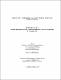## Minsum sink location and evacuation problem on dynamic cycle networks2018
##### Authors
Bashar, Md Khairul
University of Lethbridge. Faculty of Arts and Science
##### Publisher
Lethbridge, Alta. : Universtiy of Lethbridge, Department of Mathematics and Computer Science
##### Abstract
In this thesis, we study 1-sink location and k-sink evacuation problem on dynamic cycle networks. We consider the 1-sink location problem is to find the optimal location of the 1 sink, while the k-sink evacuation problem is to find the optimal evacuation protocol for the given locations of the k sinks. Both results minimize the sum of the evacuation times of all the supply located at the vertices to the sink/s of a given cycle network of n vertices. We present an efficient algorithm with a useful data structure that finds the optimal location of the 1 sink in O(n) time when the capacity of the edges are uniform. If the edges have arbitrary capacities, we solve the problem in O(nlogn)\$ time by an extension of the data structure. We also propose an O(n) time algorithm to solve the k-sink evacuation problem with uniform edge capacity.
##### Keywords
dynamic cycle networks , evacuation problem , facility location problems , minsum sink , sink location , Dissertations, Academic